# If Value is Greater Than A Certain Value

IF function is frequently used in Excel worksheet to return you expect “true value” or “false value” based on the logical test result. If you want to see if a value in one cell is greater than a specific value, you can use IF function to build a formula with logical test argument is “value>certain value”, IF function can return either true result or false result based on the test result.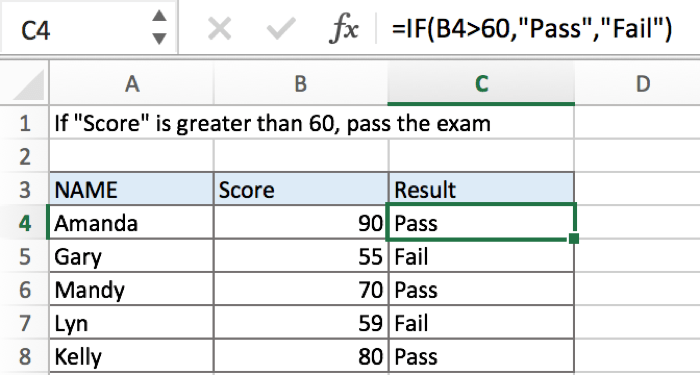In above example, in column “Score”, if cell value is greater than 60, then “Pass” is saved in “Result”; if cell value is not reached 60, “Fail” is saved in “Result”.

## FORMULA

To test if cell equals a certain text string, the generic formula is:

=IF(A1>X,”true value”,”false value”)

Formula in this example:

=IF(B4>60,”Pass”,”Fail”)

## EXPLANATION

In this example, for a student, if his score is greater than 60, that means he passed the exam, and we will add comment “Pass” in “Result” column for students who pass the exam; on the other side, for those who failed exam with a score lower than 60, we will add comment “Fail” in result. To return proper value based on a comparison, IF function can handle case like this effectively.

IF function allows you to create a logical comparison between your value and reference value (for example “A1>0”), and set true value and false value what you expect to return as test results. IF function returns one of the two results based on logical comparison result.

Syntax: IF(logica_test,[value_if_true],[value_if_false])

To test if B4 is greater than 60, we can directly create a logical comparison B4>60. There is no need to add double quotes “” to quote number 60 like B4>”60”, if you test B4=”ABC” then “ABC” should be enclosed into double quotes.

In this example, value in B4 is 90, 90>60 is true, so logical test B4>60 returns true.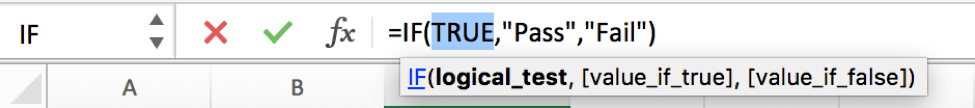As the result is True, IF function retrieves the value from “value if true” argument, in this case it is a text “Pass”, so “Pass” is displayed in C5. But for B5, B5 is 55 which a value lower than 60, so IF retrieves False value “Fail” and return “Fail” as result.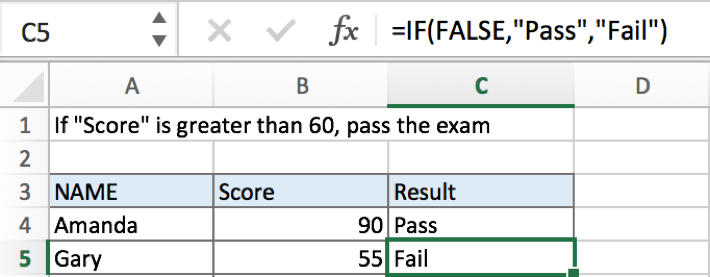In IF, true value and false value can be set as empty string “”, a text, a number or a formula, you can set what you expect to these two values, see examples below:

a.If value is not greater than 60, returns nothing by IF function.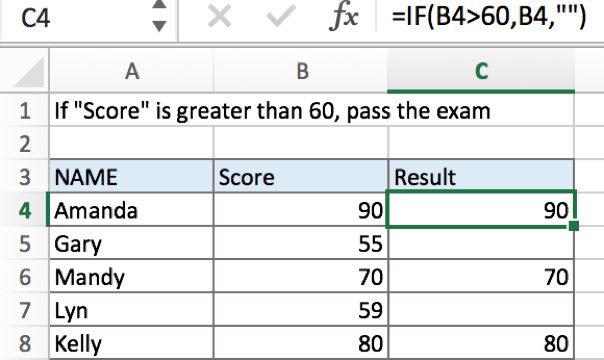b.If value is greater than 60, returns the value by IF function.c.If value is greater than 60, returns the value which is equal to “value-60” by IF function.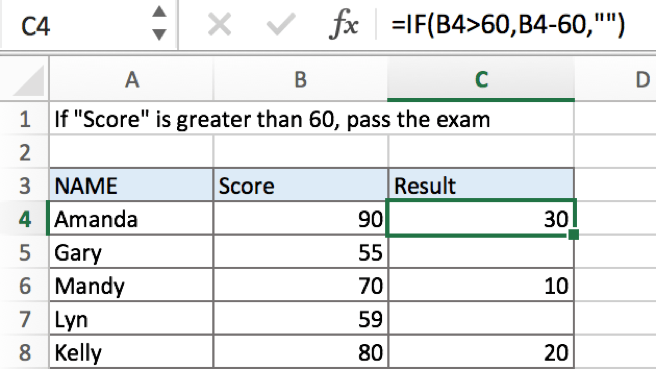### Related Functions

• Excel IF function
The Excel IF function perform a logical test to return one value if the condition is TRUE and return another value if the condition is FALSE. The IF function is a build-in function in Microsoft Excel and it is categorized as a Logical Function.The syntax of the IF function is as below:= IF (condition, [true_value], [false_value])….